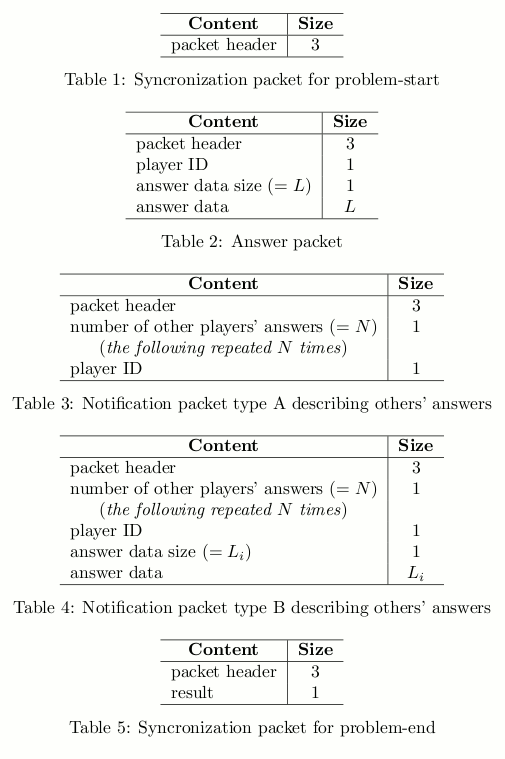시간 제한 메모리 제한 제출 정답 맞은 사람 정답 비율
1 초 512 MB 0 0 0 0.000%

## 문제

ICPC (Internet Contents Providing Company) is working on a killer game named Quiz Millionaire Attack. It is a quiz system played over the Internet. You are joining ICPC as an engineer, and you are responsible for designing a protocol between clients and the game server for this system. As bandwidth assigned for the server is quite limited, data size exchanged between clients and the server should be reduced as much as possible. In addition, all clients should be well synchronized during the quiz session for simultaneous play. In particular, much attention should be paid to the delay on receiving packets.

To verify that your protocol meets the above demands, you have decided to simulate the communication between clients and the server and calculate the data size exchanged during one game.

When each problem phase starts, the server sends a synchronization packet for problem-start to all the players, and begins a polling process. Every 1000 milliseconds after the beginning of polling, the server checks whether it received new answers from the players strictly before that moment, and if there are any, sends a notification to all the players:

• If a player hasn't submitted an answer yet, the server sends them a notification packet type $A$ (describing others' answers) about the newly received answers.
• If a player is one of those who submitted the newly received answers, the server sends them a notification packet type $B$ (describing others' answers) about all the answers submitted by other players (excluding this player's own answer) strictly before that moment.
• If a player has already submitted an answer earlier, the server sends them a notification packet type $B$ (describing others' answers) about the newly received answers.

Note that, in all above cases, notification packets (both types $A$ and $B$) must contain information about at least one player, and otherwise a notification packet will not be sent.

When $20\,000$ milliseconds have passed after sending the synchronization packet for problem-start, the server sends notification packets of type $A$ or $B$ if needed, and then sends a synchronization packet for problem-end to all the players, to terminate the problem.

Players are able to answer the problem after receiving the synchronization packet for problem-start and before receiving the synchronization packet for problem-end. Answers are sent using an answer packet.

The packets referred above have the formats shown by the following tables.## 입력

The input consists of at most $40$ test cases.

Each test case begins with a line consisting of two integers $M$ and $N$ ($1 \le M, N \le 100$), denoting the number of players and problems, respectively. The next line contains $M$ non-negative integers $D_0, D_1, \ldots, D_{M - 1}$, denoting the communication delay between each player and the server (players are assigned IDs ranging from $0$ to $M - 1$, inclusive). The communication delay is the same in both directions.

Then follow $N$ blocks describing the submissions for each problem. Each block begins with a line containing an integer $L$ ($0 \le L \le M$) denoting the number of players that submitted an answer for that problem. Each of the following $L$ lines gives the answer from one player, described by three fields $P$, $T$, and $A$ separated by whitespaces. Here, $P$ is an integer denoting the player ID, $T$ is an integer denoting the time elapsed from the reception of synchronization packet for problem-start to the submission on the player's side, and $A$ is an alphanumeric string denoting the player's answer, which has length between $1$ and $9$, inclusive. Those $L$ lines may be given in an arbitrary order.

You may assume that all answer packets will be received by the server within $19\,999$ milliseconds (inclusive) after sending synchronization packet for problem-start.

The input is terminated by a line containing two zeros.

## 출력

For each test case, print $M + 1$ lines with two integers on each line. On the first line, print the total size of the data sent and received by the server, separated by a whitespace. In the next $M$ lines, print the data size sent and received by each player, separated by a whitespace, in ascending order of player IDs. Print a blank line between two consecutive test cases.

## 예제 입력 1

3 2
1 2 10
3
0 3420 o
1 4589 o
2 4638 x
3
1 6577 SUZUMIYA
2 7644 SUZUMIYA
0 19979 YASUZUMI
4 2
150 150 150 150
4
0 1344 HOGEHOGE
1 1466 HOGEHOGE
2 1789 HOGEHOGE
3 19100 GEHO
2
2 1200 SETTEN
3 700 SETTEN
0 0


## 예제 출력 1

177 57
19 58
19 57
19 62

253 70
13 58
13 58
24 66
20 71